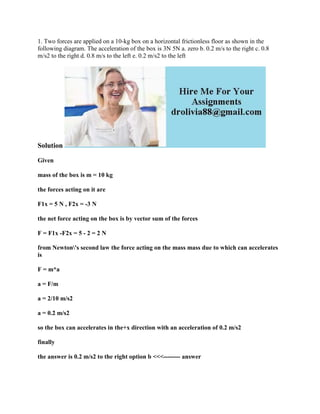Seu SlideShare está sendo baixado. ×

# 1- Two forces are applied on a 10-kg box on a horizontal frictionless.docx

Anúncio
Anúncio
Anúncio
Anúncio
Anúncio
Anúncio
Anúncio
Anúncio
Anúncio
Anúncio
AnúncioCarregando em…3
×

1 de 2 Anúncio

# 1- Two forces are applied on a 10-kg box on a horizontal frictionless.docx

1. Two forces are applied on a 10-kg box on a horizontal frictionless floor as shown in the following diagram. The acceleration of the box is 3N 5N a. zero b. 0.2 m/s to the right c. 0.8 m/s2 to the right d. 0.8 m/s to the left e. 0.2 m/s2 to the left
Solution
Given
mass of the box is m = 10 kg
the forces acting on it are
F1x = 5 N , F2x = -3 N
the net force acting on the box is by vector sum of the forces
F = F1x -F2x = 5 - 2 = 2 N
from Newton\'s second law the force acting on the mass mass due to which can accelerates is
F = m*a
a = F/m
a = 2/10 m/s2
a = 0.2 m/s2
so the box can accelerates in the+x direction with an acceleration of 0.2 m/s2
finally
the answer is 0.2 m/s2 to the right option b <<<-------- answer
.

1. Two forces are applied on a 10-kg box on a horizontal frictionless floor as shown in the following diagram. The acceleration of the box is 3N 5N a. zero b. 0.2 m/s to the right c. 0.8 m/s2 to the right d. 0.8 m/s to the left e. 0.2 m/s2 to the left
Solution
Given
mass of the box is m = 10 kg
the forces acting on it are
F1x = 5 N , F2x = -3 N
the net force acting on the box is by vector sum of the forces
F = F1x -F2x = 5 - 2 = 2 N
from Newton\'s second law the force acting on the mass mass due to which can accelerates is
F = m*a
a = F/m
a = 2/10 m/s2
a = 0.2 m/s2
so the box can accelerates in the+x direction with an acceleration of 0.2 m/s2
finally
the answer is 0.2 m/s2 to the right option b <<<-------- answer
.

Anúncio
Anúncio

### 1- Two forces are applied on a 10-kg box on a horizontal frictionless.docx

1. 1. 1. Two forces are applied on a 10-kg box on a horizontal frictionless floor as shown in the following diagram. The acceleration of the box is 3N 5N a. zero b. 0.2 m/s to the right c. 0.8 m/s2 to the right d. 0.8 m/s to the left e. 0.2 m/s2 to the left Solution Given mass of the box is m = 10 kg the forces acting on it are F1x = 5 N , F2x = -3 N the net force acting on the box is by vector sum of the forces F = F1x -F2x = 5 - 2 = 2 N from Newton's second law the force acting on the mass mass due to which can accelerates is F = m*a a = F/m a = 2/10 m/s2 a = 0.2 m/s2 so the box can accelerates in the+x direction with an acceleration of 0.2 m/s2 finally the answer is 0.2 m/s2 to the right option b <<<-------- answer